# Dot Plots in R: Quick Start Guide with Real Life Dataset

Dot plot is a type of data visualization that displays individual data points as dots along an axis.

To create a dot plot, use the dotchart(data, labels=) function, where data is a “numeric vector”, and “labels” is a vector of labels for each point. It helps compare values of different categories, spot trends, and identify outliers.

Here is the step-by-step guide:

## Step 1: Install the necessary libraries

Install ggplot2 and tidyverse package for this project. If you have not installed it, then you can install it using this code:

``````install.packages("ggplot2")

install.packages("tidyverse")``````

For this project, we will use the tcs_stock.csv dataset. You can also find this dataset on Kaggle.

``````tcs_data <- read.csv("./DataSets/tcs_stock.csv")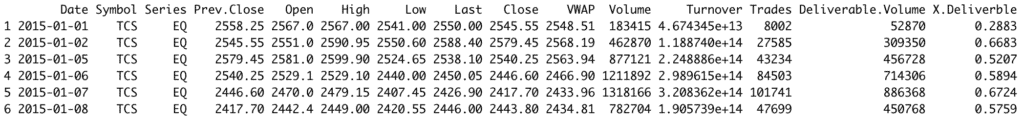## Step 3: Creating the Dot Chart

Let’s visualize the closing prices over time using a dot chart.

``````library(ggplot2)

# Sorting data by Date to ensure it's plotted chronologically
tcs_data_sorted <- tcs_data[order(as.Date(tcs_data\$Date, format="%Y-%m-%d")),]

# Create the dot chart
dotchart(tcs_data_sorted\$Close, labels = tcs_data_sorted\$Date,
main="TCS Stock Closing Prices Over Time",
xlab="Closing Price",
ylab="Date")``````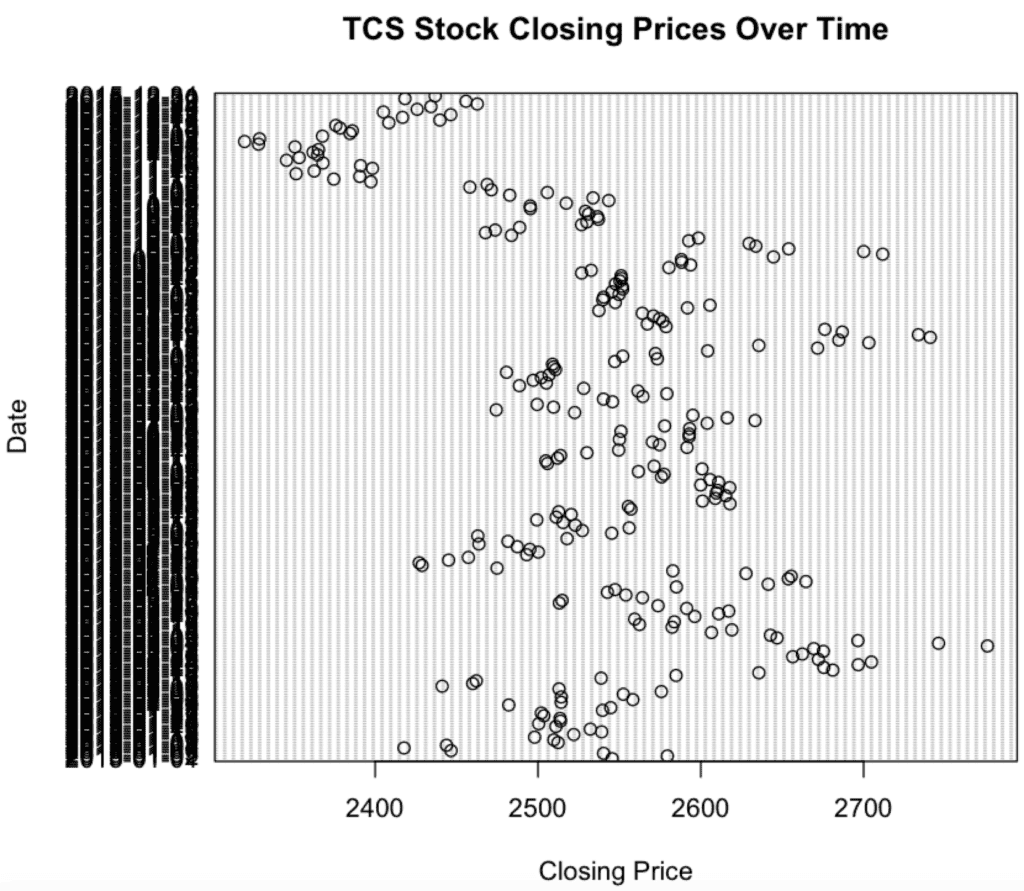You can see many dates, so the y-axis labels are crowded.

To make it more appealing, you could consider plotting only a subset of the data, adjusting the plot dimensions, or changing the label display frequency.

## Step 5: Fine-tuning

### Changing Point Color

You can change the color of the dots using the col parameter:

``````dotchart(tcs_data_sorted\$Close, labels = tcs_data_sorted\$Date,
main="TCS Stock Closing Prices Over Time",
xlab="Closing Price",
ylab="Date",
col="blue")
``````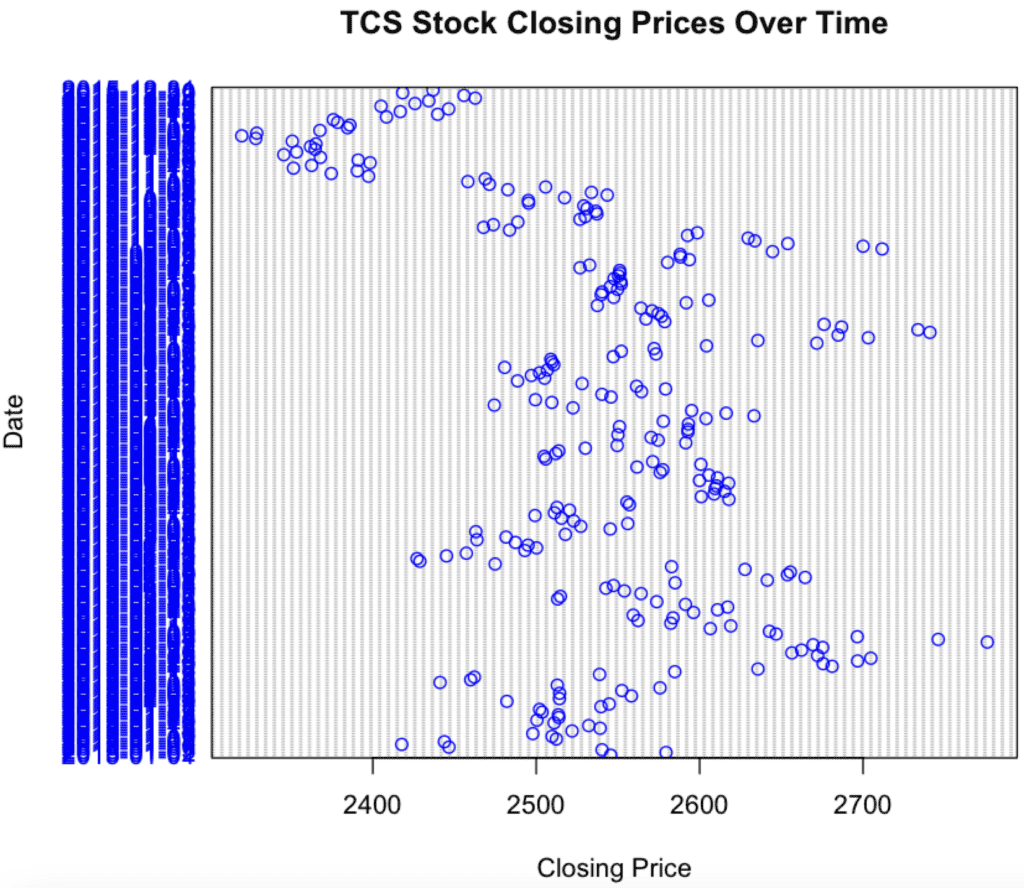### Changing Point Character

You can change the plot character of a dot plot using a pch argument.

``````dotchart(tcs_data_sorted\$Close, labels = tcs_data_sorted\$Date,
main="TCS Stock Closing Prices Over Time",
xlab="Closing Price",
ylab="Date",
pch=4) # Using a cross
``````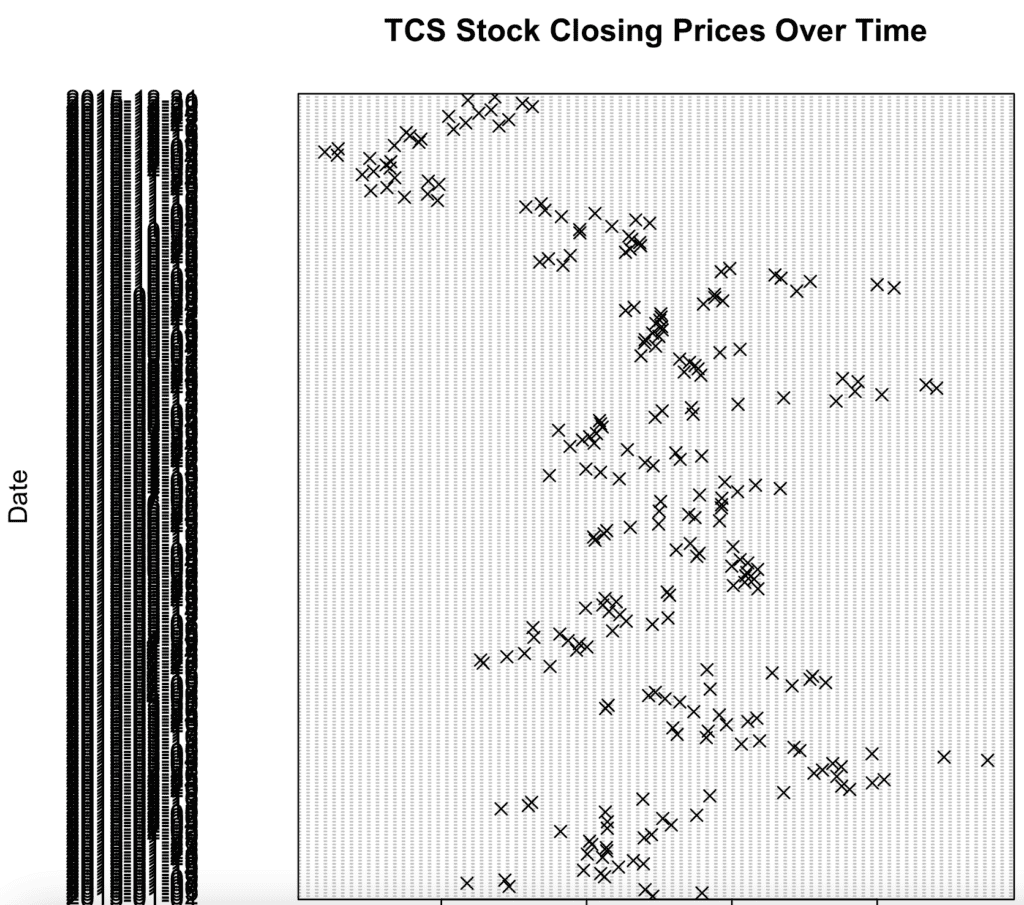### Changing Label Display Frequency

If there are too many dates and it’s crowded, you can choose to display only every nth label:

``````library(ggplot2)

# Sorting data by Date to ensure it's plotted chronologically
tcs_data_sorted <- tcs_data[order(as.Date(tcs_data\$Date, format="%Y-%m-%d")),]

every_nth <- 10 # Display every 10th label
displayed_labels <- c(rep("", every_nth-1), tcs_data_sorted\$Date)[1:nrow(tcs_data_sorted)]

dotchart(tcs_data_sorted\$Close, labels = displayed_labels,
main="TCS Stock Closing Prices Over Time",
xlab="Closing Price",
ylab="Date")``````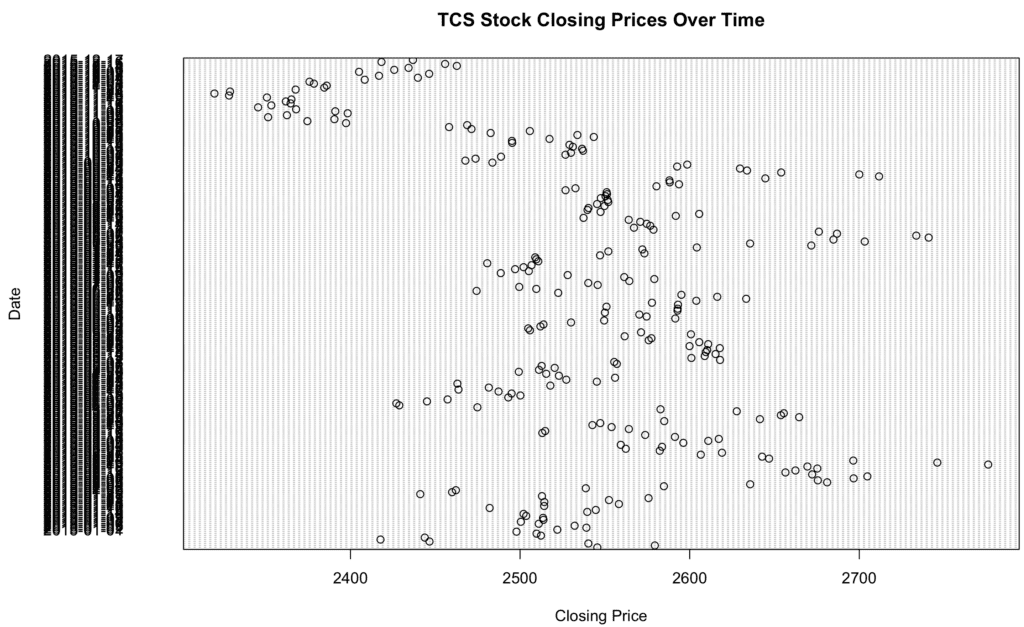## Dot plot by group

In real-life projects, we often plot by group to analyze data thoroughly.

To demonstrate, I will assume that we want to create a dot plot of the closing prices of TCS stock, grouped by year. This will help us see the distribution of closing prices within each year.

``````library(ggplot2)

# Sorting data by Date to ensure it's plotted chronologically
tcs_data_sorted <- tcs_data[order(as.Date(tcs_data\$Date, format="%Y-%m-%d")),]

tcs_data\$Year <- format(as.Date(tcs_data\$Date, format="%Y-%m-%d"), "%Y")

tcs_data_sorted <- tcs_data[order(tcs_data\$Year, as.Date(tcs_data\$Date, format="%Y-%m-%d")),]

# Create a color palette
palette <- rainbow(length(unique(tcs_data_sorted\$Year)))

dotchart(tcs_data_sorted\$Close, labels = tcs_data_sorted\$Date,
groups = tcs_data_sorted\$Year,
main = "TCS Stock Closing Prices Grouped by Year",
xlab = "Closing Price",
ylab = "Date",
color = palette[tcs_data_sorted\$Year])
legend("topright", legend = unique(tcs_data_sorted\$Year), fill = palette, title = "Year")``````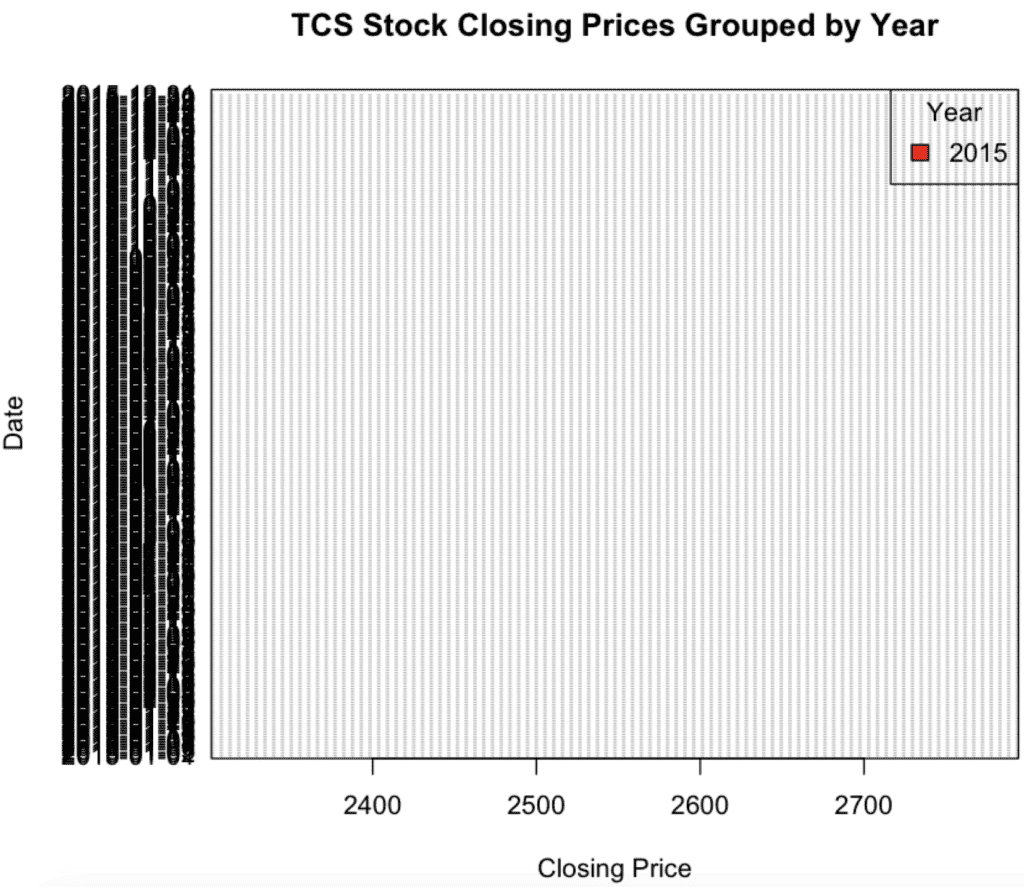## Dumbbell dot plot

A dumbbell dot plot (or dumbbell chart) is a visualization used to compare two data points for different categories side-by-side.

The two data points are typically represented as dots connected by a line, resembling a dumbbell.

The geom_dumbbell() function comes from the ggalt package. So, you need to install this library.

``````install.packages("ggalt")
``````

Now, you can create a dumbbell dot plot.

``````library(ggplot2)
library(ggalt)

ggplot(tcs_data, aes(x=Open, xend=Close, y=Date)) +
geom_dumbbell(size=1.5, color="#555555",
point.colour.l = "red", point.colour.r = "blue",
point.size.l = 3, point.size.r = 3) +
labs(title="Comparison of Opening and Closing Prices for TCS Stock",
x="Stock Price",
y="Date") +
theme_minimal()``````

Output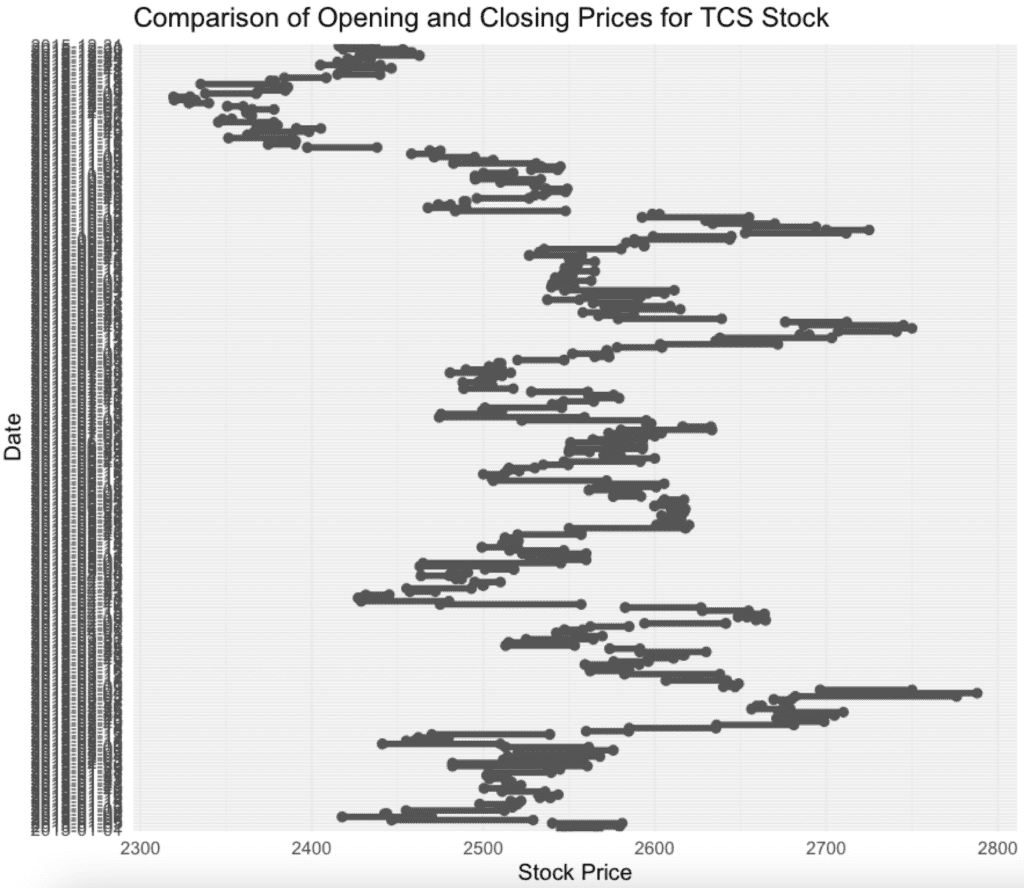## Conclusion

While dotchart() offers a simple way to create dot plots, it doesn’t have the extensive customization capabilities that ggplot2 offers.

However, for quick and simple plots, it can be quite handy.